# Extended Gaussian Image

### Manolis Kamvysselis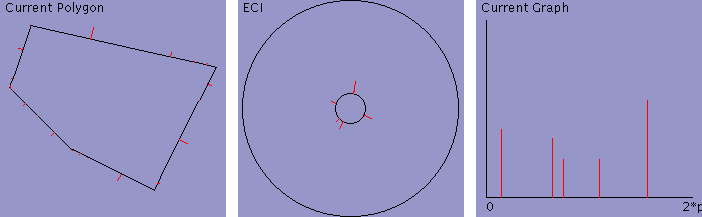1. Introduction Abstract Prior Work Motivation 2. Algorithm Overview Moving Weights Matching Weights 3. Implementation Program Using the program Source Code 4. Results Pictures Types of morphing Future Work

# Introduction

## Abstract

Morphing techniques that have been developed to progressively transform one two-dimensional image to another are usually pixel based and morph a source to a target by interpolating pixel values based on constraints specified by the user.

In this work, we are using a model-based approach to propose a solution to morphing a two-dimensional polygon into another. Our model of an object will be its Extended Circular Image representation, the two-dimensional equivalent of the Extended Gaussian Image. This approach will work only for convex polygons, for which the ECI is unique.

To morph non-convex polygons, one would have to separate them into convex components and morph each separately, or use an extended version of the ECI that would apply to non-convex polygons. This morphing method can be applied to convex polyhedra using the Extended Gaussian Image, and similarly be extended to non-convex polyhedra.

## Prior work

This term, I was working on a model-based three-dimensional morphing project for Computer Graphics.

The algorithm I developed for that project was simply matching polygons in a three-dimensional world in a nearest-neighbor fashion, and growing unmatched polygons from zero-area polygons on edges of other polygons.

The results of this approach were often quite satisfactory both graphically and mathematically, the polygons indeed traveling the way I hoped they would. However, the algorithm never assumed that the source or target objects were connected, and sometimes broke the connectivity which should have been preserved.

## Motivation

Having explored the unconnected case, I decided to explore the other extremity, where we assume that all polygons are connected. Including the additional constraint that those closed objects are convex, we can use the Extended Gaussian Image representation of an object to uniquely define it in 3D.

The problem therefore becomes that of moving the weights of the EGI on the unit sphere to redistribute them such that the EGI of the source polyhedron becomes that of the target, while keeping the center of mass at the origin (so that all intermediate objects are closed themselves).

This approach allows us to get around the problem of matching two objects of different connectivity by letting the inverse EGI function create faces of arbitrary shape, our program having specified only their size and normal direction by moving the weights on the three dimensional sphere.

The current algorithms for computing the inverse EGI being incremental, we are optimistic about this approach since the difference in connectivity and shape between two morphing steps will generally be small, allowing a faster computation of the inverse EGI.

# The Algorithm

The current two-dimensional algorithm is

1. First computing the ECI of the source and of the target polygons
2. Then matching source and target normals on the ECI circle creating source-target normal pairs
3. It then linearly interpolates weights and angles between normal pairs to derive the ECI of intermediate steps
4. Finally, it reconstructs the convex polygon corresponding to the ECI obtained by interpolating the normals.

## Moving Weights

Many approaches are possible for transforming the source normals to the target normals. Each corresponds to a different way of picturing the problem to solve. All approaches must make sure that the weights have their center of mass at the center of the unit circle at every intermediate step, such that convexity is preserved.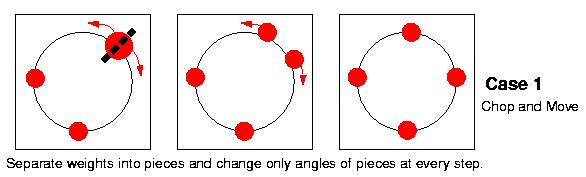One could picture the normals as physical weights on a sphere and thus want to simply interpolate angles of the normals, unable to take weight from one normal to another across the sphere. This approach will break each source weight into many chunks and it will then move those pieces of constant weight around the sphere until they recombine into the target ECI.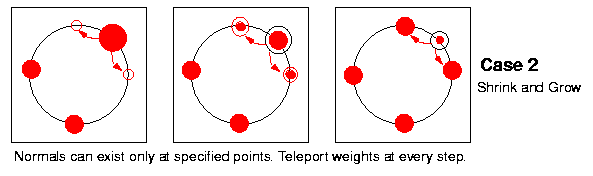Alternatively, one could consider the angles of the normals as the only places on the sphere where a weight could appear, as liquid containers all connected to each other, the weight being the quantity of liquid that we would pour into each container. We'll then simply transfer weights between normals at every step.

### General Case

We'll describe the general case as a combination of those two basic movements described above. Every normal will be allowed to move on the sphere, while distributing its weight to other normals and collecting weight from them. One can integrate both movements by matching initial and final angle and weight for every normal increment, and linearly interpolating both angle and weight. One has to then prove that the center of mass of the increments is the center of the ECI circle, to guarantee that every intermediate step will be a closed polygon.

Except from how much the angles will change and how much the weights will change, one has to make more decisions, such as which normals are affected by which others. Many choices are possible, ranging from the case where every normal is affected by and affects only the normals closest on its right and left (could be only two, or all those needed to sum up the normal's weight), to the case where every normal affects and is affected by all the other normals.

## Matching Weights

In our approach, each normal is compared with all other normals, and a cost c(i,j) of transforming every source to every target is calculated as follows: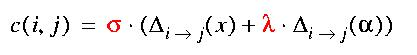where Delta(a) is the minimum angle difference between two normals (cycling around 2*pi if necessary), and Delta(w) is the weight difference between the normals. Both deltas are scaled to fit a [0..1] range, such that matching is independent of the coordinate system.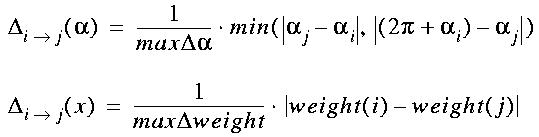Moreover, we use a lambda factor to multiply one of the two differences (here, the angle), such that a change in angle costs more than an equivalent change in weight, so that we can emphasize the properties we'd like to see preserved in our morphing.

Those costs are then entered into a function that guarantees that the center of mass of the ECI is preserved throughout the morphing process, and that intermediate polygons will also be closed. I owe this function to Panayiotis (Peter) Kamvysselis (pkamvyss@mit.edu), my older brother, with whom I discussed this interesting problem of matching normals on a unit sphere for morphing, and whose ideas and opinions were very helpful. We calculate the source normal portion corresponding to the part of the i-th normal that goes to the j-th source, by scaling the i-th normal by w(i->j) below, and the target normal portion received by the jth target from the ith source is obtained by scaling the jth target by w(j<-i) below. The function used is: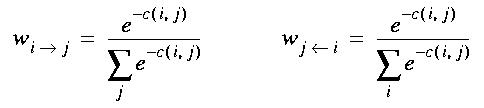This function allows for each normal to be morphed into only one other normal, if the match is very good, or contribute slightly to all other normals, if no good match exists. The appearance of the intermediate objects therefore depends on the quality of the existing matches, according to the criteria specified by the lambda above, but also on the variance 1/sigma of the function, which appears as a scale factor of the total cost c(i,j).

# Implementation

## Using the program

### To morph two polygons

• Enter the source polygon (press "Clear Source" then click once for every vertex then "Set Source")
• Enter the target polygon in the same fashion
• Press Set (above the Morph button). This calculates the increments.
• Move the scrollbar to choose morph percentage. This simply does the interpolation.

### Parameters

• Percentage
• Allows more precision than the scrollbar for choosing morph percentage.
• Total Cost
Sigma in the formula below. Compensates for individual scaling.
• Angle Cost
Lambda in the formula below. Cost of a change in angle when change in weight costs 1.• Synchronize
Automatically adjusts total cost such that: sigma*(...+lambda) = 100

### Buttons

• Clear Source/Target
• Clears the temporary data of the polygon and lets you enter points
• Set Source/Target
Reads in the temporary data drawn by the user. Calculates ECI.
• Center Source/Target
Translates the data such that bounding box and panel share center
• Scale Source/Target
Scales the data such that source and target perimeters are the same.
• Revert Source/Target
Replaces temp data with stored data.

## Code

The Java byte code is available at MM*.class, and the source is at MM*.java.

The following links will lead you to the source code of individual files of the code of the program. Feel free to use part or all of it, while giving credit to the author (Manolis Kamvysselis -- manoli@mit.edu -- http://web.mit.edu/manoli/www/).